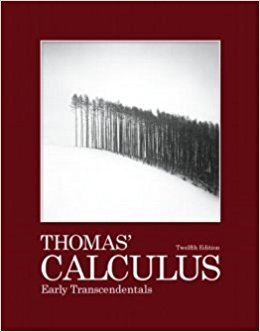×

×

# Solutions for Chapter 13: Vector-Valued Functions and Motion in Space## Full solutions for Thomas' Calculus Early Transcendentals | 12th Edition

ISBN: 9780321588760Solutions for Chapter 13: Vector-Valued Functions and Motion in Space

Solutions for Chapter 13
4 5 0 237 Reviews
28
1
##### ISBN: 9780321588760

This expansive textbook survival guide covers the following chapters and their solutions. Chapter 13: Vector-Valued Functions and Motion in Space includes 32 full step-by-step solutions. This textbook survival guide was created for the textbook: Thomas' Calculus Early Transcendentals, edition: 12. Thomas' Calculus Early Transcendentals was written by and is associated to the ISBN: 9780321588760. Since 32 problems in chapter 13: Vector-Valued Functions and Motion in Space have been answered, more than 32979 students have viewed full step-by-step solutions from this chapter.

Key Calculus Terms and definitions covered in this textbook
• Constant term

See Polynomial function

• Coordinate(s) of a point

The number associated with a point on a number line, or the ordered pair associated with a point in the Cartesian coordinate plane, or the ordered triple associated with a point in the Cartesian three-dimensional space

• Degree

Unit of measurement (represented by the symbol ) for angles or arcs, equal to 1/360 of a complete revolution

• Demand curve

p = g(x), where x represents demand and p represents price

• Dihedral angle

An angle formed by two intersecting planes,

• Distance (on a number line)

The distance between real numbers a and b, or |a - b|

• Finite sequence

A function whose domain is the first n positive integers for some fixed integer n.

• Half-life

The amount of time required for half of a radioactive substance to decay.

• Leaf

The final digit of a number in a stemplot.

• Linear programming problem

A method of solving certain problems involving maximizing or minimizing a function of two variables (called an objective function) subject to restrictions (called constraints)

• Logarithmic form

An equation written with logarithms instead of exponents

• Negative angle

Angle generated by clockwise rotation.

• NINT (ƒ(x), x, a, b)

A calculator approximation to ?ab ƒ(x)dx

• Pie chart

See Circle graph.

• Polar coordinate system

A coordinate system whose ordered pair is based on the directed distance from a central point (the pole) and the angle measured from a ray from the pole (the polar axis)

• Semiminor axis

The distance from the center of an ellipse to a point on the ellipse along a line perpendicular to the major axis.

• Standard position (angle)

An angle positioned on a rectangular coordinate system with its vertex at the origin and its initial side on the positive x-axis

• Variable (in statistics)

A characteristic of individuals that is being identified or measured.

• Variance

The square of the standard deviation.

• Zero vector

The vector <0,0> or <0,0,0>.